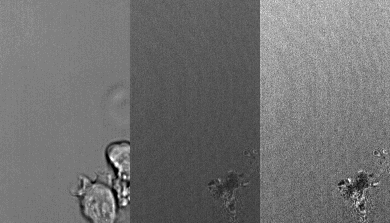One of the ways to solve flickering or changes in intensity in a movie is to float the stack.

Left:   brightfield.
Middle:   reflection raw data
Right:   reflection after float function applied.This is how we floated the stack:

```//---------------------------------------------------------
//Written by Kenton Arkill [underwaterginge@GMAIL.COM]
//Floating means that each image has the same mean pixel value and the same standard deviation.
//I find it really useful as it means the same threshold can often be used on all the images. Here is my macro,
//it floats the stack to the values of the first slice (so make sure it is a good image):

macro "Float the stack"{
run("32-bit");
setSlice(1);     // reference slice; could be set to something other than 1
getRawStatistics(nPixels, meanref, min, max, stdref, histogram);
for (i = 1; i <= nSlices; i++) {
setSlice(i);
getRawStatistics(nPixels, meani, min, max, stdi, histogram);
run("Subtract...", "value="+meani+" slice");
run("Divide...", "value="+stdi+" slice");
run("Multiply...", "value="+stdref+" slice");
}
run("Enhance Contrast", "saturated=0.35");  // could change to zero
setSlice(1);
}

```

<--back